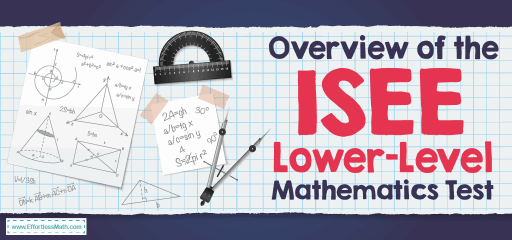# Overview of the ISEE Lower Level Mathematics TestThe Lower Level ISEE is an independent school entrance exam developed by the Educational Records Bureau (ERB) for children in grades 5 and 6. Students can take the Lower Level ISEE on paper or computer. This test is 2 hours and 20 minutes long.

The Lower Level ISEE consists of five sections:

• Verbal Reasoning: 34 questions- 20 minutes
• Quantitative Reasoning: 38 questions-35 minutes
• Reading Comprehension: 25 questions-25 minutes
• Mathematics Achievement: 30 questions-30 minutes
• Essay: 1 prompt- 30 minutes

Except for the Essay, all questions are multiple-choice with four answer options. In each section, there are randomly distributed experimental questions that may be used for later versions of the test. These experimental questions are not graded.

Mathematical skills are tested in Quantitative Reasoning and Mathematics Achievement parts of ISEE Lower Level. The ERB follows the standards set by the NCTM to determine how the ISEE tests a student’s ability in mathematics.

## How is the structure of the Quantitative Reasoning of the ISEE Lower Level Test?

The Quantitative Reasoning section focuses on critical thinking and problem-solving skills. There are 38-word problems out of which 35 are scored, and 3 are without points.

## How is the structure of the Mathematics Achievement of the ISEE Lower Level Test?

There are 30 questions in the Mathematics Achievement section of the ISEE Lower Level. The students have 30 minutes to answer the math questions. The Mathematics Achievement section is aligned with the grade-level standards, so it includes topics that students have learned in school. These topics involve:

### Numbers and Operations

• Understand numbers and ways of representing numbers
• Use all four mathematical operations (subtract, add, multiply, and divide) accurately
• Make reasonable estimations using decimals and fractions
• Accurately compute subtraction and addition using fractions and decimals
• Use properties of the operations and numbers
• Find equivalent forms of decimals, fractions, and percent

### Algebra

• Identify, extend, and find rules for relations, and patterns
• Identify, extend patterns, and compare
• Use rules that can contain variables

### Geometry

• Determine characteristics and describe geometric objects
• Describe and classify basic 2- and 3-dimensional figures
• Understand and use coordinate grids, including the transformation of geometric figures
• Understand the concepts of symmetry, congruency, and similarity

### Measurement

• Understand and determine measurable attributes of objects
• Find the perimeter and area of irregular polygons and regular
• Develop strategies to find the surface area and volume of rectangular solids
• Convert between units in both metric systems and customary
• Identify tools and units associated with capacity, length, weight, temperature, and time

### Data Analysis & Probability

• Collect, interpret, display, and make predict data
• Calculate average, median, mode, and range of a set of data
• Find the probability of a simple event

## Do you get a formula sheet on theISEE Lower Level Mathematics Test?

In the ISEE Lower Level math test you must memorize all the necessary formulas.

## Isthe ISEE Lower Level Test hard?

The ISEE Lower Level test is difficult, but if you prepare well for this test, you can succeed in this test.

## Can you use a calculator on the ISEE Lower Level Mathematics Test?

No, you are not allowed to use a calculator on the ISEE Lower Level Mathematics test.

## How is theISEE Lower Level Test scored?

The students will receive a scaled score from 760 to 940 for the math section.

## The Best Books to Ace the ISEE Lower Level Test

### What people say about "Overview of the ISEE Lower Level Mathematics Test - Effortless Math: We Help Students Learn to LOVE Mathematics"?

No one replied yet.

X
23% OFF

Limited time only!

Save Over 23%

SAVE \$5

It was \$21.99 now it is \$16.99